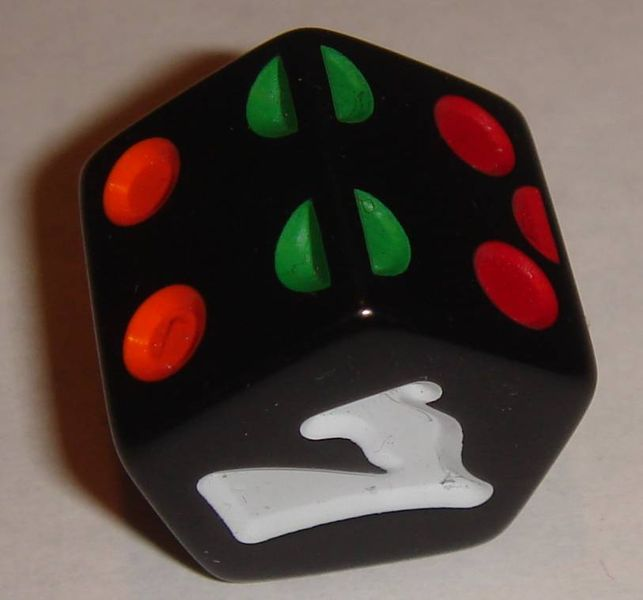# Dice sums 2

Probability Level 4Say now that we have 4 distinct dice each with 7 faces labelled $1,2,3,4,5,6,7$, and I roll all 4 simultaneously. Again let $d_{1}$ be the number that comes up on the first dice, $d_{2}$ be the number that comes up on the second dice, $d_{3}$ be the number that comes up on the third dice and $d_{4}$ be the number that comes up on the fourth dice. In how many ways can I roll the 4 dice in this way so that 3 divides $d_{1} + d_{2} + d_{3} + d_{4}$?

For the easier version, see dice sums 1.

×